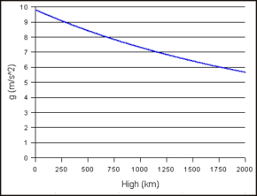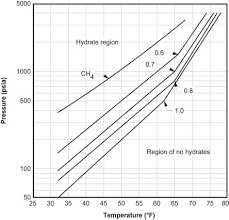## How to Calculate and Solve for Gravitational Acceleration | GravityThe image above represents gravitational acceleration.

To compute for gravitational acceleration, three essential parameters are needed and these parameters are Gravitational Constant (G), Mass of the Earth (mEand Radius of the Earth (R).

The formula for calculating gravitational acceleration:

a = GmE /

Where:

a = Gravitational Acceleration
G = Gravitational Constant
mE = Mass of the Earth
R = Radius of the Earth

Let’s solve an example;
Find the Gravitational acceleration when the gravitational constant is 6.67E-11, the mass of the earth is 12 and the radius of the earth is 21.

This implies that;

G = Gravitational Constant = 6.67E-11
mE = Mass of the Earth = 12
R = Radius of the Earth = 21

a = GmE /
a = (6.67e-11)(12) / (21)²
a = 8.004e-10 / 441
a = 1.81e-12

Therefore, the gravitational acceleration is 1.81e-12 m/s.

## How to Calculate and Solve for Separator Gas Gravity in a Fluid | The Calculator EncyclopediaThe image above represents separator gas gravity.

To compute for the separator gas gravity, four essential parameters are needed and these parameters are API Gravity (°API), Actual Separator Temperature (°Rankine) (Tsep), Actual Separator Pressure (Psep) and Gas Gravity at Actual Separator Psep and Tsepg).

The formula for calculating the separator gas gravity:

γgs = γg[1 + 5.912(10-5)(°API)(Tsep – 460)log(Psep / 114.7)]

Where:

γgs = Separator Gas Gravity
°API = API Gravity
Tsep = Actual Separator Temperature (°Rankine)
Psep = Actual Separator Pressure
γg = Gas Gravity at Actual Separator Psep and Tsep

Let’s solve an example;
Find the separator gas gravity with an API Gravity of 21, actual separator temperature of 18, actual separator pressure of 14 and gas gravity at actual separator of 32.

This implies that;

°API = API Gravity = 21
Tsep = Actual Separator Temperature (°Rankine) = 18
Psep = Actual Separator Pressure = 14
γg = Gas Gravity at Actual Separator Psep and Tsep = 32

γgs = γg[1 + 5.912(10-5)(°API)(Tsep – 460)log(Psep / 114.7)]
γgs = 32[1 + 5.912(10-5)(21)(18 – 460)log(14 / 114.7)]
γgs = 32 [1 + 5.912(10-5)(21)(18 – 460) log(0.1220)]
γgs = 32 [1 + 5.912(10-5)(21)(-442) log(0.1220)]
γgs = 32 [1 + 5.912(10-5)(21) (-442) (-0.9134)]
γgs = 32 [1 + 0.501]
γgs = 32 [1.501]
γgs = 48.039

Therefore, the separator gas gravity is 48.039.